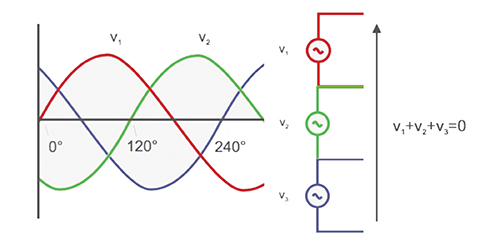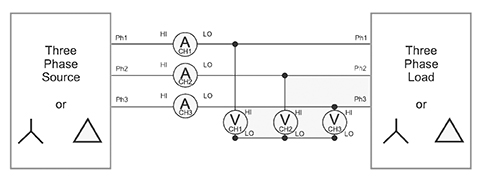# Basic three-phase power measurements explained

Although single-phase electricity is used to supply common domestic and office electrical appliances, three-phase alternating current (ac) systems are almost universally used to distribute electrical power and to supply electricity directly to higher power equipment.

This technical article describes the basic principles of three-phase systems and the difference between the different measurement connections that are possible.

• Three-phase systems
• Wye or Star connection
• Delta connection
• Wye and Delta comparison
• Power measurements
• Single-phase wattmeter connection
• Single-phase three-wire connection
• Three-phase three-wire connection (two wattmeter method)
• Three-phase three-wire connection (three wattmeter method)
• Blondel’s Theorem: number of wattmeters required
• Three-phase, four-wire connection
• Configuring measurement equipment

## Three-Phase Systems

Three-phase electricity consists of three AC voltages of identical frequency and similar amplitude. Each AC voltage phase is separated by 120° from the other (Figure 1).

This system can be represented diagrammatically by both waveforms and a vector diagram (Figure 2).

Why use three-phase systems? For two reasons:

1. The three vector-spaced voltages can be used to create a rotating field in a motor. Motors can thus be started without the need for additional windings.
2. A three-phase system can be connected to a load such that the number of copper connections required (and thus the transmission losses) is one-half of what they would otherwise be.

Consider three single-phase systems each supplying 100 W to a load (Figure 3). The total load is 3 × 100 W = 300 W.  To supply the power, 1 amp flows through 6 wires, and there are thus 6 units of loss.

Alternatively, the three supplies can be connected to a common return, as shown in Figure 4. When the load current in each phase is the same, the load is said to be balanced. With the load balanced, and the three currents phase-shifted by 120° from each other, the sum of the current at any instant is zero, and there is no current in the return line.

In a three-phase 120° system, only 3 wires are required to transmit the power that would otherwise require 6 wires. One-half of the copper is required, and the wire transmission losses will be halved.

## Wye or Star Connection

A three-phase system with a common connection is normally drawn as shown in Figure 5 and is known as a “wye” or “star” connection.

The common point is called the neutral point. This point is often grounded at the supply for safety reasons. In practice, loads are not perfectly balanced, and a fourth neutral wire is used to carry the resultant current.

The neutral conductor may be considerably smaller than the three main conductors if allowed by local codes and standards.Figure 6. The sum of the instantaneous voltage at any time is zero.

## Delta Connection

The three single-phase supplies discussed earlier could also be connected in series. The sum of the three 120° phase shifted voltages at any instant is zero. If the sum is zero, then both end points are at the same potential and may be joined together.

The connection is usually drawn as shown in Figure 7 and is known a delta connection after the shape of the Greek letter delta, Δ.

## Wye and Delta Comparison

The Wye configuration is used to distribute power to everyday single-phase appliances found in the home and office. Single-phase loads are connected to one leg of the wye between line and neutral. The total load on each phase is shared out as much as possible to present a balanced load to the primary three-phase supply.

The wye configuration can also supply single- or three-phase power to higher power loads at a higher voltage. The single-phase voltages are phase-to-neutral voltages. A higher phase-to-phase voltage is also available as shown by the black vector in Figure 8.

The delta configuration is most often used to supply higher power three-phase industrial loads. Different voltage combinations can be obtained from one three-phase delta supply, however, by making connections or “taps” along the windings of the supply transformers.

In the US, for example, a 240-V delta system may have a split-phase or center-tapped winding to provide two 120 V supplies (Figure 9).

The center-tap may be grounded at the transformer for safety reasons. 208 V is also available between the center tap and the third “high leg” of the delta connection.

### Power Measurements

Power is measured in ac systems using wattmeters.  A modern digital sampling wattmeter, such as any of the Tektronix power analyzers, multiplies instantaneous samples of voltage and current together to calculate instantaneous watts and then takes an average of the instantaneous watts over one cycle to display the true power.

A wattmeter will provide accurate measurements of true power, apparent power, volt-amperes reactive, power factor, harmonics and many others over a broad range of wave shapes, frequencies, and power factor.

For the power analyzer to give good results, you must be able to correctly identify the wiring configuration and connect the analyzer’s wattmeters correctly.

### Single-Phase Wattmeter Connection

Only one wattmeter is required, as shown in Figure 10. The system connection to the voltage and current terminals of the wattmeter is straightforward. The voltage terminals of the wattmeter are connected in parallel across the load, and the current is passed through the current terminals which are in series with the load.

### Single-Phase Three-Wire Connection

In this system, shown in Figure 11, the voltages are produced from one center-tapped transformer winding, and all voltages are in phase. This system is common in North American residential applications, where one 240 V and two 120 V supplies are available and may have different loads on each leg.

To measure the total power and other quantities, connect two wattmeters as shown in Figure 11 below.

### Three-phase Three-Wire Connection (Two Wattmeter Method)

Where three wires are present, two wattmeters are required to measure total power. Connect the wattmeters as shown in Figure 12. The voltage terminals of the wattmeters are connected phase to phase.

### Three-phase Three-Wire Connection (Three Wattmeter Method)

Although only two wattmeters are required to measure total power in a three-wire system as shown earlier, it is sometimes convenient to use three wattmeters. In the connection shown in Figure 13, a false neutral has been created by connecting the voltage low terminals of all three wattmeters together.Figure 13. Three-phase, three-wire (three wattmeter method: set analyzer to three-phase, four-wire mode.)

The three-wire, three-wattmeter connection has the advantages of indicating the power in each phase (not possible in the two-wattmeter connection) and phase to neutral voltages.

### Blondel’s Theorem: Number of  Wattmeters Required

In a single-phase system, there are just two wires. Power is measured using a single wattmeter. In a three-wire system, two wattmeters are required as shown in Figure 14.

In general, the number of wattmeters required equals the number of wires minus one.

### Proof for a three-wire wye system

The instantaneous power measured by a wattmeter is the product of the instantaneous voltage and current samples.

• Wattmeter 1 reading = i1 (v1 – v3)
• Wattmeter 2 reading = i2 (v2 – v3)
• Sum of readings W1 + W2 = i1v1 – i1v3 + i2v2 – i2v3 = i1v1 + i2v2 – (i1 + i2) v3
• (From Kirchoff’s law: i1 + i2 + i3 = 0, so i1 + i2 = -i3)
• 2 readings W1 + W2 = i1v1 + i2v2 + i3v3 = total instantaneous watts.

## Three-Phase, Four-Wire Connection

Three wattmeters are required to measure total watts in a four-wire system. The voltages measured are the true phase-to-neutral voltages. The phase-to-phase voltages can be accurately calculated from the phase-to-neutral voltages’ amplitude and phase using vector mathematics.

A modern power analyzer will also use Kirchoff’s law to calculate the current flowing in the neutral line.

## Configuring Measurement Equipment

For a given number of wires, N, N-1 wattmeters are required to measure total quantities such as power. You must make sure you have a sufficient number of (3 wattmeter method) channels, and connect them properly.

Modern multi-channel power analyzers will calculate total or sum quantities such as watts, volts, amps, volt-amperes and power factor directly using appropriate built-in formulas. The formulas are selected based on the wiring configuration, so setting the wiring is critical to get good total power measurements. A power analyzer with vector mathematics capability will also convert phase-to-neutral (or wye) quantities to phase-to-phase (or delta) quantities.

The factor √3 can only be used to convert between systems or scale the measurements of only one wattmeter on balanced, linear systems.

Understanding wiring configurations and making proper connections is critical to performing power measurements. Being familiar with common wiring systems, and remembering Blondel’s Theorem will help you get the connections right and results you can rely upon.

## References

The Fundamentals of Three-phase Power Measurements – Application Note by Tektronix

The wattmeter is an instrument for measuring the electric power (or the supply rate of electrical energy) in watts of any given circuit. Electromagnetic wattmeters are used for measurement of utility frequency and audio frequency power; other types are required for radio frequency measurements.  Source:  Wikipedia

Edvard Csanyi is an electrical engineer, programmer, and founder of Electrical Engineering Portal. Highly specialized for design of LV high power busbar trunking (<6300A) in power substations, buildings, and industry fascilities. Designing of LV/MV switchgears. Professional in AutoCAD programming and web-design. Present on Google+ Website: http://www.csanyigroup.com